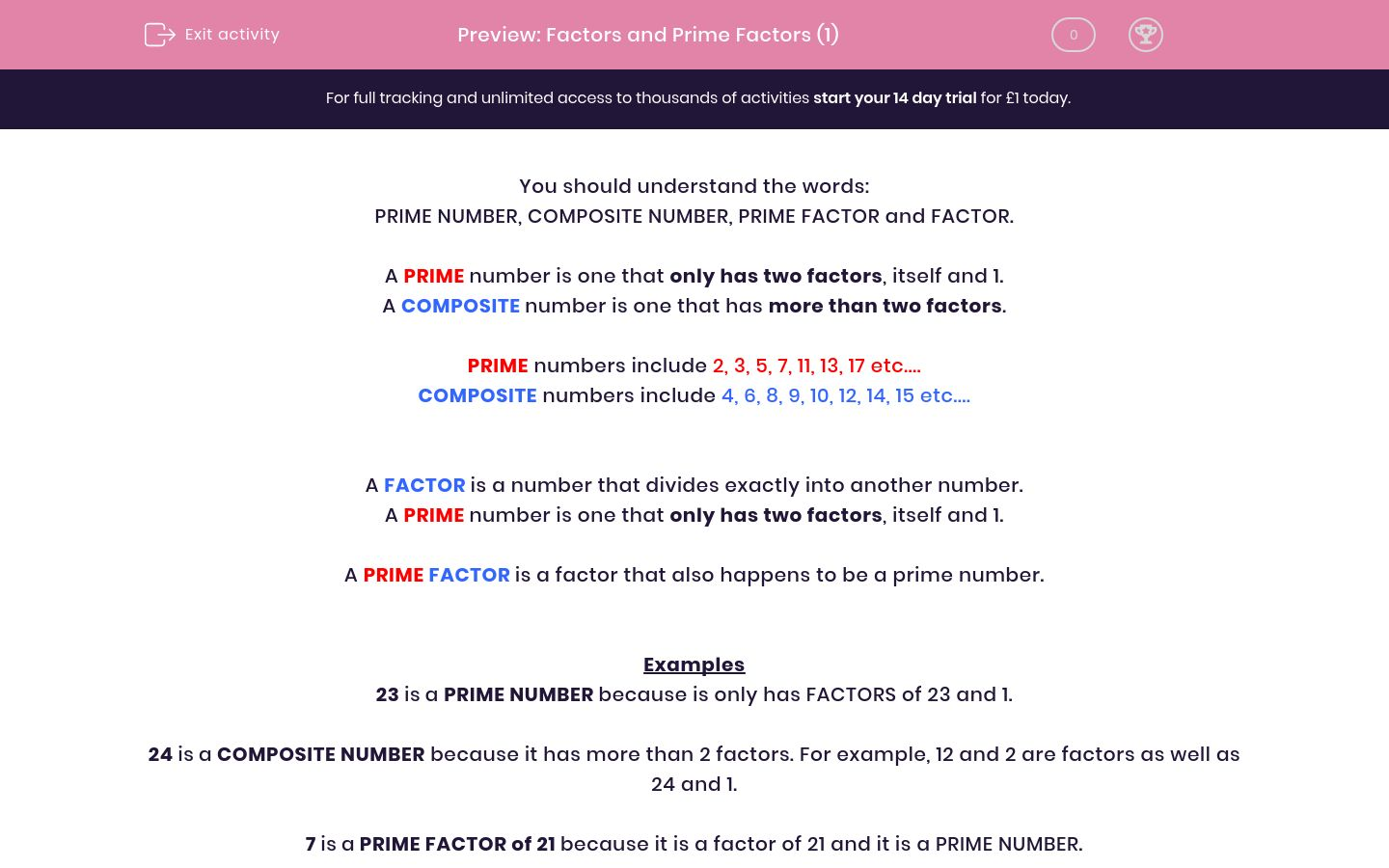# Factors and Prime Factors (1)

In this worksheet, students must complete the sentences using the correct vocabulary. Prime Numbers, Prime Factors and Composite Numbers should be understood.Key stage:  KS 2

Curriculum topic:   Maths and Numerical Reasoning

Curriculum subtopic:   Factors and Multiples

Difficulty level:#### Worksheet Overview

You should understand the words:

PRIME NUMBER, COMPOSITE NUMBER, PRIME FACTOR and FACTOR.

A PRIME number is one that only has two factors, itself and 1.

A COMPOSITE number is one that has more than two factors.

PRIME numbers include 2, 3, 5, 7, 11, 13, 17 etc....

COMPOSITE numbers include 4, 6, 8, 9, 10, 12, 14, 15 etc....

A FACTOR is a number that divides exactly into another number.

A PRIME number is one that only has two factors, itself and 1.

A PRIME FACTOR is a factor that also happens to be a prime number.

Examples

23 is a PRIME NUMBER because is only has FACTORS of 23 and 1.

24 is a COMPOSITE NUMBER because it has more than 2 factors. For example, 12 and 2 are factors as well as 24 and 1.

7 is a PRIME FACTOR of 21 because it is a factor of 21 and it is a PRIME NUMBER.

### What is EdPlace?

We're your National Curriculum aligned online education content provider helping each child succeed in English, maths and science from year 1 to GCSE. With an EdPlace account you’ll be able to track and measure progress, helping each child achieve their best. We build confidence and attainment by personalising each child’s learning at a level that suits them.

Get started